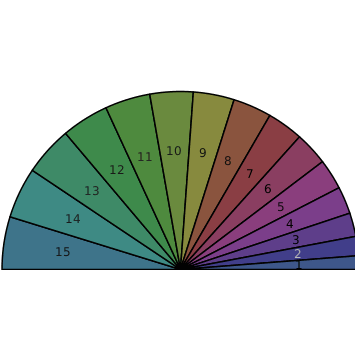Laplacian - Maple Help

Student[VectorCalculus]

 Laplacian
 compute the Laplacian of a function or a vector fieldCalling Sequence Laplacian(f, c) Laplacian(F)Parameters

 f - algebraic expression c - (optional) specify the coordinate system F - (optional) vector field or Vector-valued procedure; a vector fieldDescription

 • The Laplacian(f) calling sequence computes the Laplacian of the function f in the current coordinate system.  If no coordinate system has been explicitly specified, the command will assume a cartesian system with coordinates the variables which appear in the expression f. This is equivalent to $\left(\mathrm{Del}·\mathrm{Del}\right)\left(f\right)$ and $\nabla ·\nabla \left(f\right)$.
 • The Laplacian(f, c) calling sequence computes the Laplacian of the function f in the coordinate system specified by the parameter c, which can be given as:
 * an indexed name, e.g., ${\mathrm{spherical}}_{r,\mathrm{\phi },\mathrm{\theta }}$
 * a name, e.g., spherical; default coordinate names will be used
 * a list of names, e.g., $\left[r,\mathrm{\phi },\mathrm{\theta }\right]$; the current coordinate system will be used, with these as the coordinate names
 The Laplacian(c) calling sequence returns the differential form of the Laplacian operator in the coordinate system specified by the parameter c.
 The Laplacian() calling sequence returns the differential form of the Laplacian operator in the current coordinate system.  If no coordinate system has been set (by a call to SetCoordinates), cartesian coordinates are assumed.
 • The Laplacian(F) calling sequence, where F is either a vector field or a Vector-valued procedure (which is interpreted as a vector field), computes the Laplacian of the vector field as follows.
 *  If the coordinate system of F is cartesian, the command maps the algebraic Laplacian onto the component functions.
 *  If F is a 3-D vector field, use the formula $\mathrm{Del}\left(\mathrm{Del}·F\right)-\mathrm{Del}\phantom{\rule[-0.0ex]{0.3em}{0.0ex}}&x\phantom{\rule[-0.0ex]{0.3em}{0.0ex}}\left(\mathrm{Del}\phantom{\rule[-0.0ex]{0.3em}{0.0ex}}&x\phantom{\rule[-0.0ex]{0.3em}{0.0ex}}F\right)$.
 *  Otherwise, map F to cartesian coordinates, apply the algebraic Laplacian to the component functions, and then map the result back to the original coordinate system of F.Examples

 > $\mathrm{with}\left({\mathrm{Student}}_{\mathrm{VectorCalculus}}\right):$
 > $\mathrm{Laplacian}\left({x}^{3}+{y}^{3}+{z}^{3}\right)$
 ${6}{}{x}{+}{6}{}{y}{+}{6}{}{z}$ (1)
 > $\mathrm{Laplacian}\left({x}^{3}+a{y}^{3},\left[x,y\right]\right)$
 ${6}{}{a}{}{y}{+}{6}{}{x}$ (2)
 > $\mathrm{Laplacian}\left(\right)$
 $\frac{{{\partial }}^{{2}}}{{\partial }{{x}}^{{2}}}\phantom{\rule[-0.0ex]{0.4em}{0.0ex}}{\mathrm{SF}}{}\left({x}{,}{y}\right){+}\frac{{{\partial }}^{{2}}}{{\partial }{{y}}^{{2}}}\phantom{\rule[-0.0ex]{0.4em}{0.0ex}}{\mathrm{SF}}{}\left({x}{,}{y}\right)$ (3)
 > $\mathrm{Laplacian}\left(f\left(r,\mathrm{θ}\right),{\mathrm{polar}}_{r,\mathrm{θ}}\right)$
 $\frac{\frac{{\partial }}{{\partial }{r}}\phantom{\rule[-0.0ex]{0.4em}{0.0ex}}{f}{}\left({r}{,}{\mathrm{\theta }}\right){+}{r}{}\left(\frac{{{\partial }}^{{2}}}{{\partial }{{r}}^{{2}}}\phantom{\rule[-0.0ex]{0.4em}{0.0ex}}{f}{}\left({r}{,}{\mathrm{\theta }}\right)\right){+}\frac{\frac{{{\partial }}^{{2}}}{{\partial }{{\mathrm{\theta }}}^{{2}}}\phantom{\rule[-0.0ex]{0.4em}{0.0ex}}{f}{}\left({r}{,}{\mathrm{\theta }}\right)}{{r}}}{{r}}$ (4)

The Laplacian in cylindrical coordinates:

 > $\mathrm{SetCoordinates}\left({\mathrm{cylindrical}}_{r,\mathrm{θ},z}\right)$
 ${{\mathrm{cylindrical}}}_{{r}{,}{\mathrm{\theta }}{,}{z}}$ (5)
 > $\mathrm{Laplacian}\left(f\left(r,\mathrm{θ},z\right)\right)$
 $\frac{\frac{{\partial }}{{\partial }{r}}\phantom{\rule[-0.0ex]{0.4em}{0.0ex}}{f}{}\left({r}{,}{\mathrm{\theta }}{,}{z}\right){+}{r}{}\left(\frac{{{\partial }}^{{2}}}{{\partial }{{r}}^{{2}}}\phantom{\rule[-0.0ex]{0.4em}{0.0ex}}{f}{}\left({r}{,}{\mathrm{\theta }}{,}{z}\right)\right){+}\frac{\frac{{{\partial }}^{{2}}}{{\partial }{{\mathrm{\theta }}}^{{2}}}\phantom{\rule[-0.0ex]{0.4em}{0.0ex}}{f}{}\left({r}{,}{\mathrm{\theta }}{,}{z}\right)}{{r}}{+}{r}{}\left(\frac{{{\partial }}^{{2}}}{{\partial }{{z}}^{{2}}}\phantom{\rule[-0.0ex]{0.4em}{0.0ex}}{f}{}\left({r}{,}{\mathrm{\theta }}{,}{z}\right)\right)}{{r}}$ (6)
 > $\mathrm{.}\left(\mathrm{Del},\mathrm{Del}\right)\left(f\left(r,\mathrm{θ},z\right)\right)$
 $\frac{\frac{{\partial }}{{\partial }{r}}\phantom{\rule[-0.0ex]{0.4em}{0.0ex}}{f}{}\left({r}{,}{\mathrm{\theta }}{,}{z}\right){+}{r}{}\left(\frac{{{\partial }}^{{2}}}{{\partial }{{r}}^{{2}}}\phantom{\rule[-0.0ex]{0.4em}{0.0ex}}{f}{}\left({r}{,}{\mathrm{\theta }}{,}{z}\right)\right){+}\frac{\frac{{{\partial }}^{{2}}}{{\partial }{{\mathrm{\theta }}}^{{2}}}\phantom{\rule[-0.0ex]{0.4em}{0.0ex}}{f}{}\left({r}{,}{\mathrm{\theta }}{,}{z}\right)}{{r}}{+}{r}{}\left(\frac{{{\partial }}^{{2}}}{{\partial }{{z}}^{{2}}}\phantom{\rule[-0.0ex]{0.4em}{0.0ex}}{f}{}\left({r}{,}{\mathrm{\theta }}{,}{z}\right)\right)}{{r}}$ (7)
 > $\mathrm{Laplacian}\left(\right)$
 $\frac{\frac{{\partial }}{{\partial }{r}}\phantom{\rule[-0.0ex]{0.4em}{0.0ex}}\left({r}{}\left(\frac{{\partial }}{{\partial }{r}}\phantom{\rule[-0.0ex]{0.4em}{0.0ex}}{\mathrm{SF}}{}\left({r}{,}{\mathrm{\theta }}{,}{z}\right)\right)\right){+}\frac{{\partial }}{{\partial }{\mathrm{\theta }}}\phantom{\rule[-0.0ex]{0.4em}{0.0ex}}\left(\frac{\frac{{\partial }}{{\partial }{\mathrm{\theta }}}\phantom{\rule[-0.0ex]{0.4em}{0.0ex}}{\mathrm{SF}}{}\left({r}{,}{\mathrm{\theta }}{,}{z}\right)}{{r}}\right){+}\frac{{\partial }}{{\partial }{z}}\phantom{\rule[-0.0ex]{0.4em}{0.0ex}}\left({r}{}\left(\frac{{\partial }}{{\partial }{z}}\phantom{\rule[-0.0ex]{0.4em}{0.0ex}}{\mathrm{SF}}{}\left({r}{,}{\mathrm{\theta }}{,}{z}\right)\right)\right)}{{r}}$ (8)
 > $F≔\mathrm{VectorField}\left(⟨{r}^{3},\frac{z}{\mathrm{θ}},\sqrt{z}⟩\right)$> $\mathrm{simplify}\left(\mathrm{Laplacian}\left(F\right)\right)$> $\mathrm{simplify}\left(\mathrm{Del}\left(\mathrm{.}\left(\mathrm{Del},F\right)\right)-\mathrm{Del}&x\left(\mathrm{Del}&xF\right)\right)$